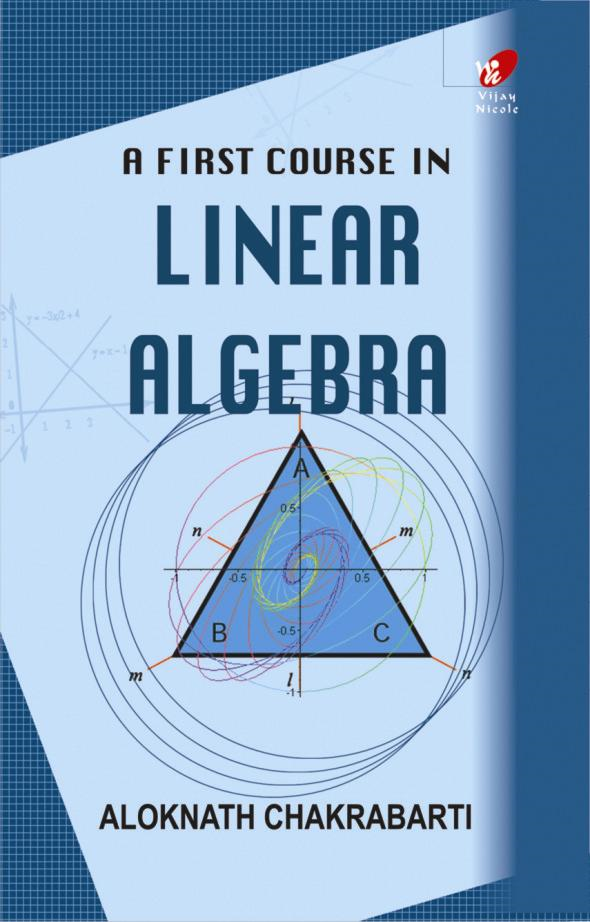A First Course in Linear Algebra
Author(s):
Subject(s):
ISBN 9789394681774
Published Date
Pages 374
Description

Linear Algebra is of extreme importance in mathematics and has applications in almost all branches of science and engineering. This book exposes you to the subject at the fundamental level as learnt by undergraduate and postgraduate students of mathematics.

Cover
Halftitle Page
Title Page
Dedication Page
Contents
Preface
1. Matrices and their Uses
Basic Definitions and Concepts
Algebraic Operations Involving Matrices
The Gaussian Method (and the Gauss-Jordan method)
The Inverse of a Square Matrix
The Gaussian Method
Exercise
2. Determinats and their Uses
Basic Definitions
Simplifications to Evaluate Determinants
Properties of Determinants
Inverse of Matrices Using Determinants—Cramer's Rule
Exercise
3. The Euclidean Vector Spaces
Euclidean n-space (Rn)
Linear Transformations from Rn to Rm
Important Results Involving Linear Transformations
Exercise
4. General Vector Spaces
Real Vector Spaces
Basis and Dimension
The Four Fundamental Spaces
Rank and Nullity
Exercise
5. Inner-Product Spaces
Inner-product in General
Orthogonality
Orthogonal and Orthonormal Basis—Gram-Schmidt Process
Least Squares Solution
Orthogonal Matrices
Exercise
6. Eigenvalue Problems and their Applications
Basic Definitions
Diagonalization of Square Matrices
Orthogonal Diagonalization
Application of Diagonalization
Some Special Results
Exercise
7. Worked Out Problems
Worked Out Problems Involving Chapter 1
Worked Out Problems Involving Chapter 2
Worked Out Problems Involving Chapter 3
Worked Out Problems Involving Chapter 4
Worked Out Problems Involving Chapter 5
Worked Out Problems Involving Chapter 6
Index